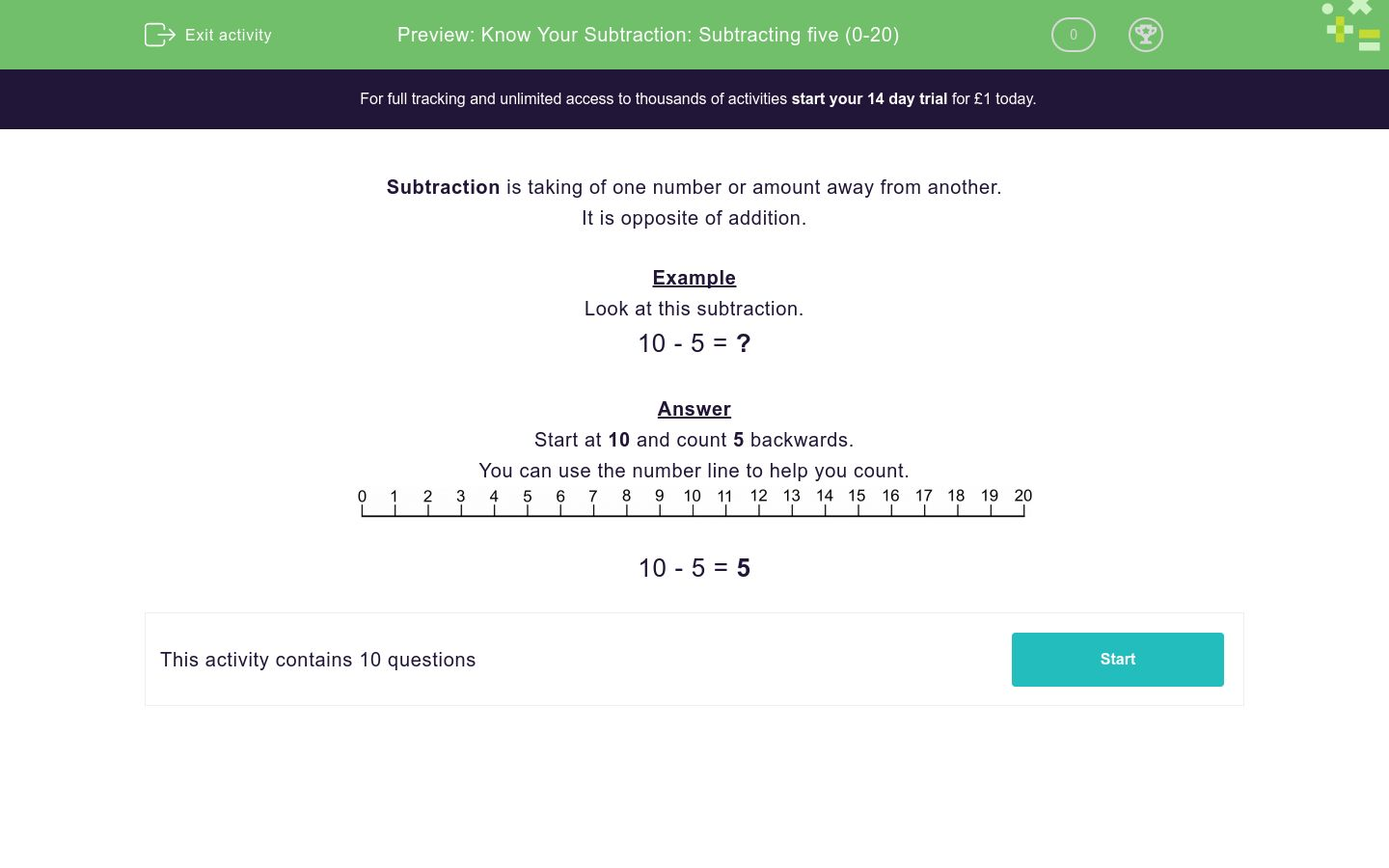# Know Your Subtraction: Subtracting five (0-20)

In this worksheet, students practise their subtraction skills using the number five.Key stage:  KS 1

Curriculum topic:   Number: Addition and Subtraction

Curriculum subtopic:   Add and Subtract to 20

Difficulty level:### QUESTION 1 of 10

Subtraction is taking of one number or amount away from another.

Example

Look at this subtraction.

10 - 5 = ?

Start at 10 and count 5 backwards.10 - 5 = 5

---- OR ----